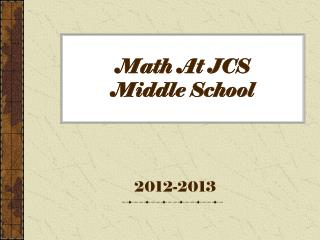DownloadDownload PresentationMath At JCS Middle School

# Math At JCS Middle School

Télécharger la présentation## Math At JCS Middle School

- - - - - - - - - - - - - - - - - - - - - - - - - - - E N D - - - - - - - - - - - - - - - - - - - - - - - - - - -
##### Presentation Transcript

1. Math At JCS Middle School 2012-2013

2. IMPORTANT!! • We all want students to be successful! • To be successful, teachers parents & students need to know what to expect.

3. Strands in Middle School Math • Numbers and Operations • Patterns and Relations • Shape and Space • Data Management and Probability

4. A Balanced Math Program has… • Warm ups • Mental Math • Whole Class Instruction • Guided Math/ Anchor Activities • Journals • Games • Project Math

5. Assessment Balanced Math = Various Assessment Methods

6. Achievement Indicators • A+ = WOW! • A = Gotitwell • B = Gotit • C = Just gotit • D = Almostthere • E = Not quitethere

7. District and Provincial Assessments • Grades 6 and 7 students write District Assessments at the end of May or beginning of June • Grade 8 students write Provincial Assessments at the beginnig of June

8. Sample Mental Math 1. 5  9 = _____ 7. 2 x 6 + 3 x 6 =_____ 2. 43  100 = ____ 8. 32 + 42 =_____ 3. 60  30 = _____ 9. \$2.98 + \$3.99=_____ 4. ½ + ¼ = _____ 10. ½ x 18= ____ 5. 3416 ÷ 2 = _____ 11. 24 x 25= ___ 6. 0.2  14 = _____ 12. 600- 291= ____

9. Multiple Choice Which equation does this diagram represent? a) 2x + 5 = 9 b) 2x – 5 = 9 c) 1x + 5 = 9 2 d) 1x - 5 = 9 2

10. Non-Calculator-Gr 7 Solve (-3) – (+2) using a drawing.

11. Non-Calculator-Gr 8 • How many ¼ litres are in 3 ½ litres?

12. Open Response * Show all work for each question* • Create a realistic word problem that is solved by multiplying 6 by 2 ½ . • Show the solution to your word problem.

13. To be Successful….. Parents can help by: • Making sure they have a good breakfast. • Making sure students get lots of rest. • Make sure they come with the proper supplies. ie. Pencils, calculator etc. • Encourage mental math strategies whenever you can. • Encourage them to do the best they can!!

14. Teamwork • Together we can make a difference!